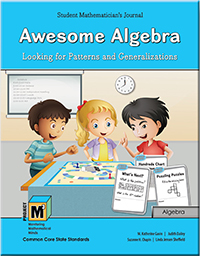# Project M³ Curriculum Units — Level 3-4

## Awesome Algebra: Looking for Patterns and GeneralizationsWe do not usually think of algebra as a topic for the elementary mathematics classroom. However, Operations and Algebraic Thinking, one of the domains created in the Common Core State Standards, illustrates the development of algebraic reasoning in elementary mathematics. The reality is that many of the mathematical explorations based on arithmetic in the early grades are actually representative of algebraic thinking and are necessary precursors for the study of more formal algebra in middle and high school. Looking for patterns, extending a pattern and making a generalization about a pattern are all part of algebraic thinking. We just have never associated the study of patterns with algebra before and the connection may not be obvious. We need to move away from thinking about algebra as the content found in a high school mathematics program such as solving quadratic equations and finding the slope of a line. This is not what the Common Core State Standards for Mathematics nor the National Council for Teachers of Mathematics are talking about as algebra at the elementary level. Rather we need to explore students’ thinking to see what might be foundational to algebraic reasoning. So we talk about algebraic thinking or reasoning as opposed to the formal study of algebra.

In our Project M3 unit, Awesome Algebra: Looking for Patterns and Generalizations, students are encouraged to study patterns and determine how they change, how they can be extended or repeated and/or how they grow. They then move beyond this to organize the information systematically and analyze it to develop generalizations about the mathematical relationships in the patterns. There is a strong focus on mathematical discourse revolving around how to verbalize a generalization.

Algebra is in many respects the generalization of arithmetic and so students are using algebraic thinking when they make generalizations. For example, in the early grades students discover that the sum of 20 + 30 is the same as the sum of 30 + 20. Soon they realize that this holds true no matter what two numbers they choose to add. When students are encouraged to make up a rule that explains this discovery they are actually using algebraic reasoning and making a generalization about an addition property. During this unit, students will be encouraged to use the idea of a variable as they think about how to represent a rule. This will help them become aware of the usefulness of a variable when representing a generalization. Our emphasis on number patterns is designed to challenge mathematically talented students by encouraging them to take a new look at basic number concepts, i.e. arithmetic, from an algebraic perspective. For example in Chapter 3, students focus on the commutative and associative properties of addition. These properties will help students develop a much stronger number sense. This, in turn, will make them better estimators and give them effective tools to perform computation mentally.